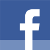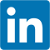# Accessing a fusion sequence with a run time defined index

published at 24.03.2018 23:05

Lets say you have a compile type defined type, like a tuple or a fusion sequence. And its easy to access, just call get<Index>(variable) and you get the reference to the types run time instance in the index. Easy. But for this you need to know which index to call at compile time. What if you get that index only at runtime? Like in the previously mentioned Model/View Interface of Qt?

In the last installment of this small series, it was all about writing a model, and hence many methods got a QModelIndex with column() and row() to then either set a value or get the value that is in that position. And row would be the nth member of a fusion adapted struct in this case. And when ever such a situation occured, a function called visit_fusion_sequence_at was called, which is what this blog post is about:

`visit_fusion_sequence_at(con[index.row()],index_array[index.column()],[&x](auto& v){assign(x,v);});`

This function takes the element to access, and the index for the nth member you wish to access. And a lambda as a callback, which calls a different function with the parameter value and a value from outside. This is how the data is exchanged.

The implementation of visit_fusion_sequence_at:

```template < typename F, typename Seq>
void visit_fusion_sequence_at(Seq & s, size_t idx, F&& fun)
{
detail::fusion_visit_impl<boost::fusion::result_of::size< Seq>::value>::visit(s, idx, std::forward< F>(fun));
}```

The call gets forwarded into a template class with a static method, similar in detail with the one shown in the first installment of this series:

```namespace detail{
template <size_t I>
struct fusion_visit_impl
{
template < typename Seq, typename F>
static void visit(Seq& s, size_t idx, F&& fun)
{
static_assert(boost::fusion::result_of::size< Seq>::value >= I,"fusion index out of bounds");
if (idx == I -1) fun(boost::fusion::get< I-1>(s));
else fusion_visit_impl< I -1>::visit(s, idx, std::forward<F>(fun));
}
};

template < >
struct fusion_visit_impl< 0 >
{
template < typename Seq, typename F>
static void visit(Seq& , size_t , F&& ) { }
};
}```

The lambda is called, once the index matches, otherwise its counting down the index recursively to test the next index. The case for 0 is specialized, so this travesty comes to an end.

Which is all fantastic, but most structs aren't that big, so a bit of unrolling this compiletime recursion into a switch could save a lot of calls to the above type. And a switch lets you map a run time value to a compile time value. So the updated implementation of visit_fusion_sequence_at looks like this:

```template
void visit_fusion_sequence_at(Seq & s, size_t idx, F&& fun)
{
switch(idx)
{
case 0:
get_by_index<0>(s,std::forward< F>(fun));
break;
...
case 9:
get_by_index<9>(s,std::forward< F>(fun));
break;
default://*/
detail::fusion_visit_impl<boost::fusion::result_of::size< Seq>::value>::visit(s, idx, std::forward(fun));
}
}```

For this to work, one needs to use std::enable_if on get_by_index, so that boost::fusion::get is only called in the version, that is enabled for when the index is smaller then the struct size, like this:

```template< size_t I, class Seq, class F >
typename std::enable_if< boost::mp11::mp_less< boost::mp11::mp_size_t< I> , boost::fusion::result_of::size< Seq>>::value,void>::type get_by_index(Seq& s, F && fun)
{
fun(boost::fusion::get< I>(s));
}

template< size_t I, class Seq, class F >
typename std::enable_if<!boost::mp11::mp_less< boost::mp11::mp_size_t< I> , boost::fusion::result_of::size< Seq>>::value,void>::type get_by_index(Seq& ,F&&){}//*/```

So by little bit of more template magic, it is achieved, that get_index_by is only calling fusion::get on the indexes allowed by the type. The default is for supporting large types with 10+ members, when actually also querying these members beyond index 9.

But then a comment on reddit pointed at mp11::mp_with_index, which can do all of the above, so the updated version of visit_fusion_sequence_at is:

```template < typename F, typename Seq>
void visit_fusion_sequence_at(Seq & s, size_t idx, F&& fun)
{
boost::mp11::mp_with_index< boost::fusion::result_of::size::value>(idx,[&](auto I){
fun(boost::fusion::get< I>(s));
});
}```

This replaces the recursive calls in detail and the switch. So the implementation becomes easy to read code, which is also much shorter and cleaner then the previous versions.

## Assigning the value...

One might wonder why the lambda above calls an assign function, rather then just doing x = v; (or v = x;). There are two reasons for this. First, types which aren't convertible directly will lead to a compilation error then, and assign can actually be overloaded and selected with enable_if. I've implemented a similar function for converting strings to the correct type a little while ago for the JSON Import in my CMS.

Right now only is_convertible and is_constructible are checked, to ensure the type b can be assigned to a:

```template< class T, class V>
typename std::enable_if< std::is_convertible< T,V >::value || std::is_constructible< T,V >::value, void>::type assign(T& to, const V& from)
{
to = from;
}

// throw error when type conversion is not possible
template< class T, class V>
typename std::enable_if< !std::is_convertible< T,V >::value && !std::is_constructible< T,V >::value, void>::type assign(T& , const V& )
{
throw std::runtime_error("impossible conversion");//*/
}```

This code would be a lot easier to write with if constexpr...

But ofc this doesn't play nice with things that don't convert automatically, like Qt types. So for these one needs to provide overloads:

```void assign(std::string &s, const QString &qs)
{
s = qs.toStdString();
}
template< class T>
void qt_assign(T& t, const QVariant& v)
{
assign(t,v.value());
}
```

As mentioned, the code often has to deal with variant, assigning to a QVariant is not a problem, but assigning from it needs to call value<T>. So I chose to write a small helper template function to do this. The overload of assign is called instead of assign, so that the error is not triggered.

Join the Meeting C++ patreon community!
This and other posts on Meeting C++ are enabled by my supporters on patreon!